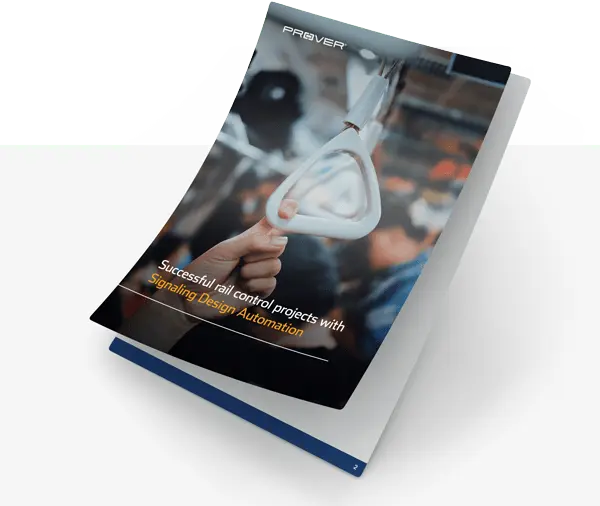If you have read the first two parts of this series (Read Part 1 here and Part 2 here), then you know that verifying an interlocking that satisfies safety requirements is not an easy task to accomplish. Due to the huge size of the state space of the interlocking, neither manual methods nor testing will get you all the way. You also know that mathematics is the place to look for methods that will. So finally we can start talking about formal verification.

As usual in science, in order to transfer the verification problem into the realms of mathematics, we make mathematical models of the parts involved: the interlocking and the safety requirements. The part of mathematics that can provide us with suitable methods and concepts is logic. What we want to know are things like the following:

When the interlocking commands a signal to show proceed, is there a locked route that begins at that signal?

We want to provide convincing, airtight, arguments showing that such statements are true; i.e. we want a proof of this. Mathematical logic is exactly the part of mathematics that can provide us with an answer. There, things like ‘truth’, ‘statement’, and ‘proof’ are given a precise meaning. The core of the matter is to make mathematics of this kind of question:

Does the truth of statement A force the truth of statement B?
Or, which luckily amounts to the same thing,
Is there a proof of statement B from statement A?

And that is exactly the kind of mathematics we need to solve the verification problem.

In formal verification, what we do is build a logical model of the interlocking – our statement A – and another model of the safety requirements – our statement B. Hence, we have managed to transform the verification problem into a mathematical problem, which means that if we are able to produce a proof of statement B from statement A, we have actually proved that the interlocking satisfies the safety requirements. We have proved that the interlocking will never enter a state where the safety requirements are violated.

So in theory, all is well. Does it work in practice?

Fortunately, it does work in practice as well. The reason is that the most important tasks can be done by computer tools. In particular, there are computer tools that can find a proof of statement B from statement A. When it comes to the modeling parts, the biggest one is the modeling of the interlocking. But also this task can be done by computer tools similar to a compiler, translating the interlocking program into a logical model while preserving all important aspects of the original program. The modeling of the requirements is usually done by hand, but that can be done in a generic part and a specific part. These are then feed into the proving tool which specializes the generic requirements to the particular interlocking that is to be verified. Thus, the generic requirements can then be reused for other interlockings, which minimizes the time spent on modeling the requirements.

This blog concludes this series of blogs answering why formal verification is the solution to the verification problem. I hope you are convinced. Or are at least curious.